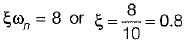Courses

# Time Domain Analysis of Control Systems - 3

## 20 Questions MCQ Test Topicwise Question Bank for Electrical Engineering | Time Domain Analysis of Control Systems - 3

Description
This mock test of Time Domain Analysis of Control Systems - 3 for Electrical Engineering (EE) helps you for every Electrical Engineering (EE) entrance exam. This contains 20 Multiple Choice Questions for Electrical Engineering (EE) Time Domain Analysis of Control Systems - 3 (mcq) to study with solutions a complete question bank. The solved questions answers in this Time Domain Analysis of Control Systems - 3 quiz give you a good mix of easy questions and tough questions. Electrical Engineering (EE) students definitely take this Time Domain Analysis of Control Systems - 3 exercise for a better result in the exam. You can find other Time Domain Analysis of Control Systems - 3 extra questions, long questions & short questions for Electrical Engineering (EE) on EduRev as well by searching above.
QUESTION: 1

### For the given series RLC circuit, damping ratio (ξ) and undamped natural frequency (ωn) are respectively given by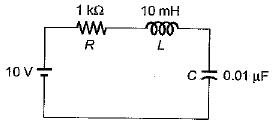Solution:

The characteristic equation of series RLC circuit is given by: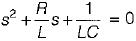Comparing with s2 + 2ξωns + ωn2 = 0, we have: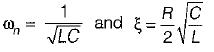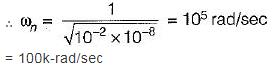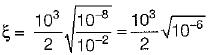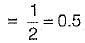QUESTION: 2

### For the unity feedback control system shown below, by what factor the amplifier gain K should be multiplied so that the damping ratio is increased from 0.3 to 0.9?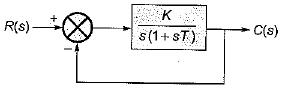Solution:

Characteristic equation of given system is
1 + G(s) H(s) = 0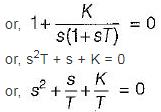Here,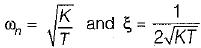For ξ1 = 0.3, gain = K1
For ξ2 = 0.9, gain = K2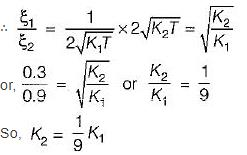QUESTION: 3

### A system is shown in figure below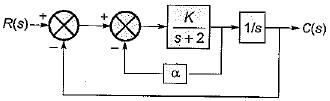What are the values of K and α respectively so that the system has a damping ratio of 0.7 and an undamped natural frequency ωn of 4 rad/sec ?

Solution:

From given block diagram, the closed loop transfer function is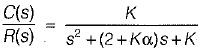Characteristic equation is,
s2 + (2 + Kα) s + K = 0
Here, ωn = -√K
and 2ξωn = (2 + kα)
Given, ωn = 4 rad/sec
∴ K = 16
Also, ξ = 0.7
∴ 2 x 0.7 x 4 = (2 + 16α)
or, (2 + 16α) = 5.6
or,
α = 3.6/16 = 0.225

QUESTION: 4

For type-2 system, the steady state error due to parabolic input is

Solution:

For parabolic input,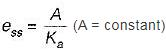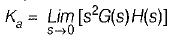= Finite for type-2 system
So, ess = Some finite value

QUESTION: 5

The open loop transfer function of a unity-feedback control system with unit step input is given by: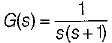The settling time of the system for 5% tolerance band is,

Solution:

Characteristic equation is: 1 + G(s) H(s) = 0 or, s2 + s + 1 = 0
Here,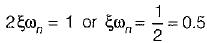For 5% tolerance band, settling time is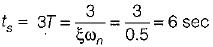QUESTION: 6

The integral of a ramp function becomes

Solution:
QUESTION: 7

Consider a unity feedback control system as shown in figure below: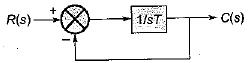The error signal e(t) for a unit ramp input for T ≥ 0 is

Solution: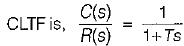Given,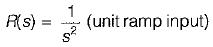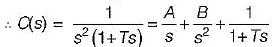Here, A = -T, 6 = 1, C = T2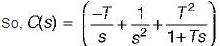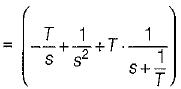Taking inverse Laplace transform on both sides, we get:
C(t) = (-T + t + Te-t/T)
∴ Error signal,
e(t) = c(t) - r(t)
= c(t) - t = - T + Te-t/T
= T(1 - e-t/T)

QUESTION: 8

A second order system has a closed loop transfer function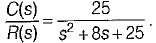If the system is initially at rest and subjected to a unit step input at t = 0, the third peak in the response will occur at

Solution: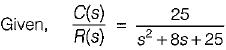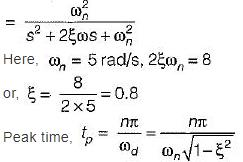For 3rd peak, n = 5
(3rd overshoot is the third peak)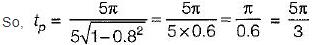QUESTION: 9

A closed servo system is represented by the differential equation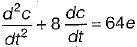where c is the displacement of the output shaft r is the displacement of the input shaft anc e = r - c.
The damping ratio and natural frequency for this system are respectively

Solution:

Given,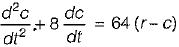or, s2C(s) + 8s C(s) = 64 [R(s) - C(s)]
or,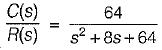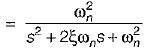Hence, ωn = 8 rad/sec
and 2ξωn = 8 or ξ = 0.5

QUESTION: 10

The poles of a closed loop control system art located at -1 ± j.
For the given system match List-I (Time domain specifications) with List - II (Values) and select the correct answer using the codes given below the lists
List - I
A. Damping ratio
B. Undamped natural frequency (rad/s)
C. Damping factor
D. Settling time (in secs)
List - II
1. 4.0
2. 1.0
3. 0.707
4. 1.414
Codes: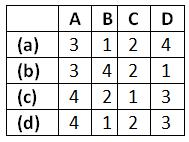Solution:

Given, poles are at s = -1 ± j ∴ Characteristic equation is
(s+1)2 + 1 =0
or, s2 + 2s + 2 = 0
Comparing above equation with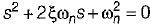we have, ωn = √2 rad/sec
and 2ξωn = 2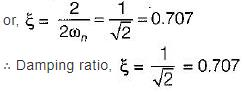Undamped natural frequency,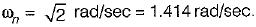Damping factor = ξωn = 1
Settling time (For 2% tolerance band) is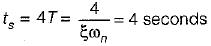Damped natural frequency is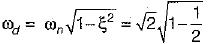= 1 rad/sec

QUESTION: 11

Consider the block diagram of a control system shown below.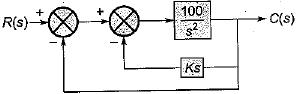What is the value of gain k such that the system is non oscillatory yet has the lowest possible settling time to step input?

Solution:

Forward gain,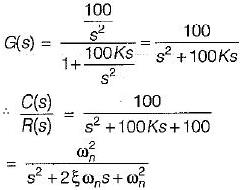Here, ωn = 10 rad/s and 2ξωn > = 100 K
or,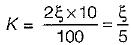For no-oscillation and to have lowest possible settling time ξ = 1.
∴ K = 1/5 = 0.2

QUESTION: 12

Assertion (A): The "dominant pole" concept is used to control the dynamic performance of the system, whereas the “insignificant poles” are used for the purpose of. ensuring that the controller transfer function can be realized by physical components.
Reason (R): if the magnitude of the real part of a pole is at least 5 to 10 times that of a dominant pole or a pair of complex dominant poles, the pole may be regarded as insignificant in so far as the transient response is concerned.

Solution: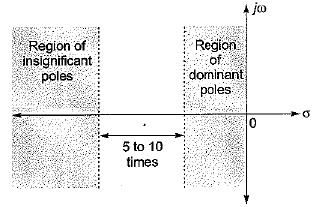Both assertion and reason are true. However, the correct explanation of assertion is that the poles having real part magnitude 5 to 10 times of the magnitude of real part of dominant poles doesn't affect the transient response (dynamic performance) of the system to a great extent due to which they are termed as insignificant poies and hence are neglected for transient stability study purpose.

QUESTION: 13

Assertion (A): The step-function input represents an instantaneous change in the reference input.
Reason (R): If the input is an angular position of a mechanical shaft, a step input represents the sudden rotation of the shaft.
(a) Both A and R are true and R is a correct explanation of A.
(b) Both A and R are true but R is not a correct explanation of A.
(c) A is true but R is false.
(d) A is false but R is true.

Solution:
QUESTION: 14

Consider the following statements related to second order control system with unit step input:
1. The right-half s-plane corresponds to negative damping.
2. Negative damping gives a response that doesn’t grows in magnitude without bound with time.
3. The imaginary axis corresponds to zero damping.
4. The maximum overshoot is often used to measure the relative stability of a control system.
5. Higher the damping in the system larger is the peak overshoot in the transient response of the system.
Which of the above statements is/are correct?

Solution:

Negative damping gives a response that grows in magnitude without bound in time. Hence, statement-2 is false.
Since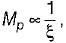therefore higher is the damping in the system, less is the peak overshoot of the system. Hence, statement-5 is not correct

QUESTION: 15

Which of the following is not true regarding addition of a pole to the forward-path transfer function?

Solution:

With addition of a pole to the forward-path transfer function, bandwidth will reduce.
Since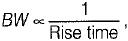therefore rise time will increase.
Since rise time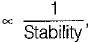therefore stability will decrease.

QUESTION: 16

For a second-order prototype system, when the undamped natural frequency is increased, the maximum overshoot of the output will

Solution:

We know that, maximum overshot is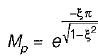Mp is independent of wn. So M∝ ξ only.

QUESTION: 17

Consider a unity feedback system shown in figure below where, K > 0 and a > 0.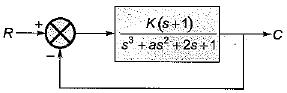What are the values of k and a respectively so that the system oscillates at a frequency of 2 rad/sec?

Solution:

Given,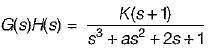Now, 1 + G(s)H(s) = 0
or, s3 + as2 + (2 + K) s + (1 + K) = 0
Routh’s array is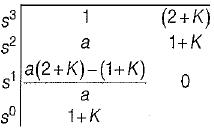For oscillation, we have: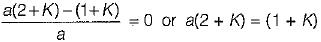Also, oscillating frequency will be given by roots of A2(s) = 0.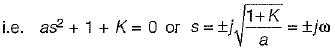Given,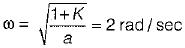We see that only option (d) is matching, i.e. for K = 2 and a = 0.75,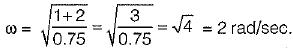QUESTION: 18

The transient response of a system is mainly due to

Solution:
QUESTION: 19

A second order system has a damping ratio ξ and undamped natural frequency of oscillation ωn respectively. The settling time at 5% of tolerance band of the system is

Solution:

For 2% tolerance band,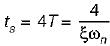For 5% tolerance band,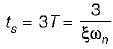QUESTION: 20

The unit impulse response of a linear time invariant second order system is
g(t) = 10e-8t sin 6t (t ≥ C)
The natural frequency and the damping ratio of the system are respectively

Solution:

Given, c(t) = g(t) = 10e-8t sin6t
Here,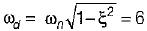and ξωn = 8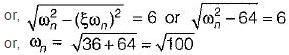= 10 rad/sec
So,